#### 期刊菜单

Simulation Analysis and Comparison of Four Voltage Doubling Rectifier Circuit
DOI: 10.12677/OJCS.2020.92005, PDF , HTML, XML, 下载: 657  浏览: 2,379

Abstract: The input voltage is the mains supply. Multisim 14 simulation software is used to simulate the sim-ple voltage doubling rectifier circuit, half-wave voltage doubling rectifier circuit, symmetrical volt-age doubling rectifier circuit, Schenkel voltage doubling rectifier circuit and full-wave voltage dou-bling rectifier circuit. The circuit structure, the effective value of output voltage, ripple and stable time about various common several voltage doubling rectifier circuits have been analyzed and compared. Then, a summary is given about merits and drawbacks as well as different applicable occasions, providing a theoretical basis for the design of high voltage circuit.

1. 引言

2. 4倍压整流电路

3. 电路仿真与分析  

3.1. 简单倍压整流电路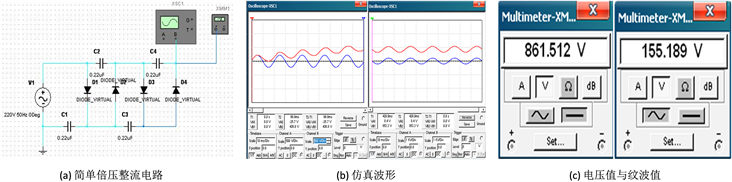Figure 1. Simulation and test results of single voltage doubling rectifier circuit

3.2. 半波倍压整流电路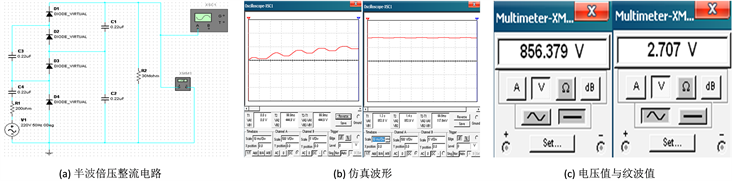Figure 2. Simulation and test results of half-wave voltage doubling rectifier circuit

3.3. 对称倍压整流电路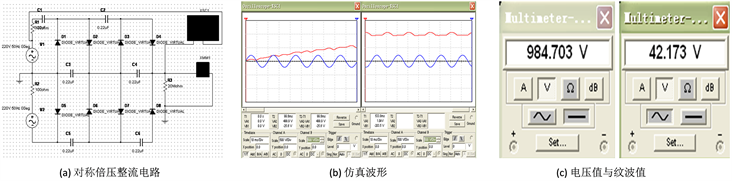Figure 3. Simulation and test results of symmetrical voltage doubling rectifier circuit

3.4. 信克尔倍压整流电路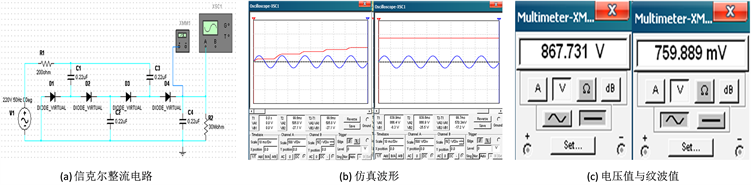Figure 4. Simulation and test results of Schenkel voltage doubling rectifier circuit

3.5. 全波倍压整流电路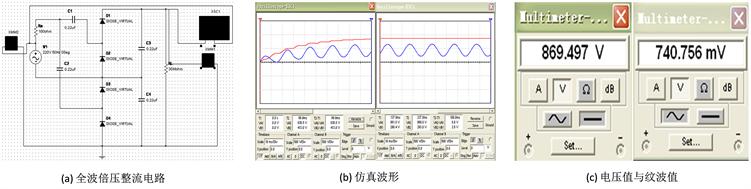Figure 5. Simulation and test results of full-wave voltage doubling rectifier circuit

4. 性能比较Table 1. Characteristic analysis and comparison of voltage doubling rectifier circuit

5. 结束语

  闫良, 闫英敏, 杨凤彪. 倍压整流电路的分析和仿真[J]. 电子设计工程, 2017, 25(8): 119-123.  赵文杰, 万成安, 郑岩. 基于倍压整流电路的空间高压电源设计[J]. 电子设计工程, 2019, 27(20): 64-69, 74.  陈鼎元, 邓吉利. 高铁中倍压整流电路的原理分析与仿真[J]. 电焊机, 2018, 48(3): 384-386.  张文昌, 刘海鹏, 孙晓钰. 单相功率倍压整流电路的建模与仿真[J]. 通信电源技术, 2019, 36(9): 33-34, 37.  徐周, 王宏华, 郭鹏. 基于倍压整流电路的高压交流电源设计[J]. 科学技术与工程, 2014, 14(13): 191-194.  刘立军. 纳米金刚石场致发射显示器驱动电路设计[D]: [硕士学位论文]. 西安: 西北大学, 2008.  银志军, 赵扬, 孙大维, 等. 倍压整流电路的仿真与分析[J]. 光电技术应用, 2006, 21(5): 71-75.